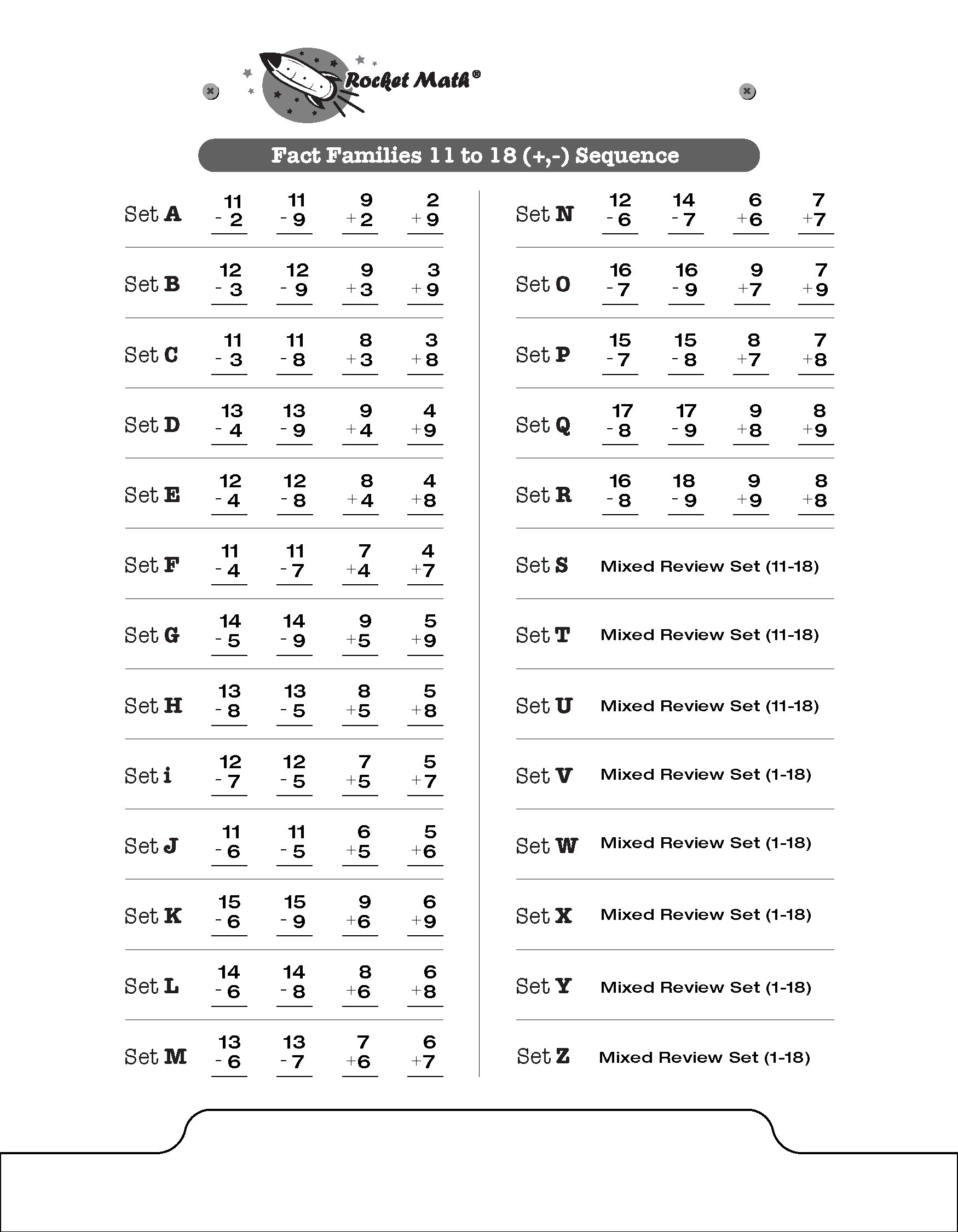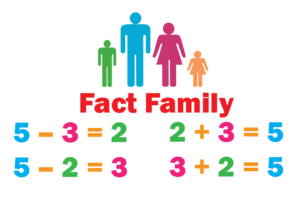## Add and Subtract Fact Families are a different way to learn facts.

A number of math programs around the country introduce math facts in families.  Now Rocket Math does too!  A fact family includes both addition and subtraction facts. This program is Part 2 of Fact Families, coming after Fact Families 1 to 10. You can see above the 18 examples of fact families taught in this program starting with Set A; 11-2, 11-9, 9+2, & 2+9.  The sheet above shows the sequence of learning facts in the new Rocket Math  program Add-Subtract Fact Families from 11 to 18.  Each set that students learn from A to R adds just one fact family to be learned, so it isn’t too hard to remember.  (That’s the Rocket Math secret ingredient!)Learning math facts in families, is gaining in popularity these days.  Logic suggests that this would be an easier way to learn.  However, the research is not definitive that this is easier or a faster way to learn facts than separating the operations and learning all addition facts first and then learning all subtraction facts.  But learning in fact families is a viable option, and I wanted to have it available for Rocket Math customers.

## Fact Families from 11 to 18 is part two and fit for second grade.

These facts come after the Add and Subtract Fact Families 10, which should be learned in first grade, so these are best for second grade.  The 25 fact families in 1s through 10s facts are just enough for one Rocket Math program.  It is a good and sufficient accomplishment for first grade.  With the 11 to 18 part for second grade there will be a lot of review.  In fact sets S through Z are all review. I have heard that some first grades prefer to keep the numbers small but to learn both addition and subtraction–so this program accomplishes that.

We  most sincerely want students to be successful and to enjoy (as much as possible) the necessary chore of learning math facts to automaticity. Please give us feedback when you use this program, Add-Subtract Fact Families 11 to 18,  letting us know how it goes for the students.